# SQL语句大全 - 看这一篇就够了

## select（选择）

``````SELECT 列名称 FROM 表名称
SELECT * FROM 表名称    --选中全部列
SELECT a,b FROM 表名称  --选中a、b``````

## distinct（去重）

``SELECT DISTINCT 列名称 FROM 表名称    --列值去重``

## where（约束）

``SELECT 列名称 FROM 表名称 WHERE 列 运算符 值``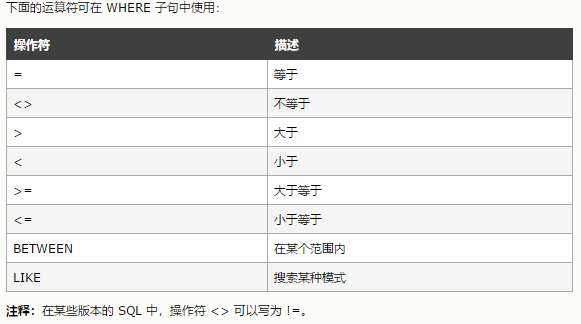## order by（排序）

``````SELECT a,b FROM table ORDER BY a;    --返回a和b列，并将结果按照a升序排序
SELECT a,b FROM table ORDER BY a,b;  --返回a和b列，并将结果按a升序排序，如a有重复，则重复部分按b升序排序
SELECT a,b FROM table ORDER BY a DESC, b ASC;--返回a和b列，并将结果按a降序排序，如a有重复，则重复部分按b升序排序``````

## insert（插入）

``````INSERT INTO 表名称 VALUES (值1, 值2,....) --插入一行数据
INSERT INTO table_name (列1, 列2,...) VALUES (值1, 值2,....) --插入指定列数据``````

## update（更新）

``UPDATE 表名称 SET 列名1=新值1, 列名2=新值2,... WHERE 列名称 = 某值    --更新多个列的数据``

## delete（删除）

``````DELETE FROM 表名称 WHERE 列名称 = 值    --删除列=值得指定行
DELETE FROM 表名称    --删除整个表的全部数据（不会删除表。表结构会被保留）``````

## like（模糊匹配）

``````SELECT * FROM table WHERE a LIKE '123%'    --返回所有a字段的值以123开头的行
SELECT * FROM table WHERE a LIKE '%123'    --返回所有a字段的值以123结尾的行
SELECT * FROM table WHERE a LIKE '%123%'   --返回所有a字段的值且包含关键字123的行``````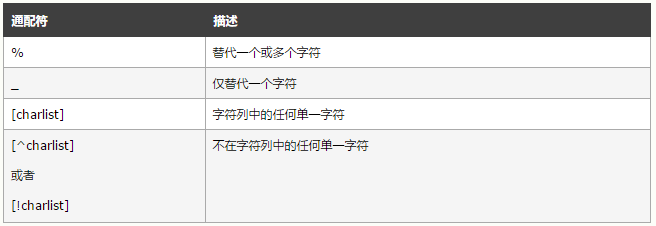``````SELECT * FROM table WHERE a LIKE 'r_d' --匹配a字段值为r*d的(*为任意一个字符)
SELECT * FROM table WHERE a LIKE '%[red]%' --匹配a字段值包含r/e/d中任意一个字符的``````

## limit（数量限制）

``````SELECT * FROM table LIMIT n    --返回前n行数据
SELECT * FROM table LIMIT m, n --返回m+1行开始往后数n条数据
SELECT * FROM table LIMIT m, -1 --返回m+1行到末尾的全部数据``````

``SELECT * FROM table LIMIT 5, 10    --返回6-15行的数据``

## in（包含）

``SELECT * FROM table WHERE a IN (value1,value2,...) --返回a值为value1,value2,...中任意一个值的行``

in的用法和python中的"variable in list"用法类似

## between（范围）

``SELECT * FROM table WHERE a BETWEED 1 AND 5 --返回a字段值在[1, 5)之间的行``

## alias（别名）

``````----表别名
--不使用别名的情况，对于复杂sql语句且名字较长的，可读性下降：
SELECT a_long_name_table_a.col_a, another_long_name_table_b.col_b
FROM a_long_name_table_a, another_long_name_table_b
WHERE a_long_name_table_a.col_a='123' AND another_long_name_table_b.col_b='456'

--使用别名的情况，sql语句简洁明了
SELECT t1.col_a, t2.col_b
FROM a_long_name_table_a AS t1, another_long_name_table_b AS t2
WHERE t1.col_a='123' AND t2.col_b='456'

----列别名
SELECT a_long_column_name AS a, another_long_column_name AS b
FROM table    --获取两个列的数据，并在结果集中列名改为a和b``````

## join（连接）

inner join：内连接，返回表1和表2满足条件（ON后面的是条件）的全部行。即取满足条件的交集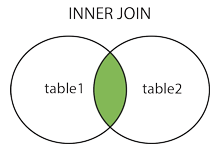``SELECT * FROM table1 INNER JOIN table2 ON table1.a=table2.b``

left join：左连接，返回表1(左表)的全部行以及表2(右表)满足条件的全部行，即取左表+交集，缺失数据用NULL表示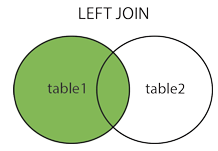``SELECT * FROM table1 LEFT  JOIN table2 ON table1.a=table2.b``

right join：右连接，返回右表全部行和左表满足条件的全部行，即右表+交集，缺失数据用NULL表示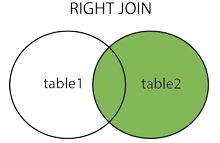``SELECT * FROM table1 RIGHT JOIN table2 ON table1.a=table2.b``

full join：全连接，返回左右表全部行，缺失数据用NULL表示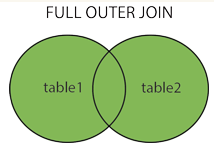``SELECT * FROM table1 FULL  JOIN table2 ON table1.a=table2.b``

## union（联合）

``````SELECT student FROM tsinghua
UNION
SELECT student FROM pku
--返回tsinghua的student字段和pku的student字段（去重）

--不想去重，使用UNION ALL

SELECT student FROM tsinghua
UNION ALL
SELECT student FROM pku
--返回tsinghua的student字段和pku的student字段（不去重）``````

## select into（选择插入）

``SELECT 字段 INTO 目标表 FROM 源表 WHERE 约束条件    --满足约束条件的行从源表拷贝到目标表``

## create（创建）

``````--创建名为dbname的数据库
CREATE DATABASE dbname

--创建一个表
CREATE TABLE 表名称
(
列名称1 数据类型 约束(可有可无),
列名称2 数据类型 约束(可有可无),
列名称3 数据类型,
....
PRIMARY KEY (主键名称)
)``````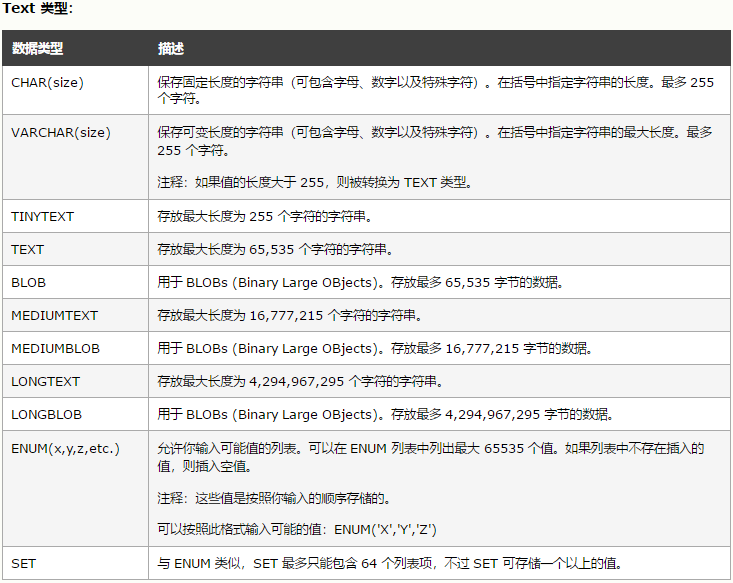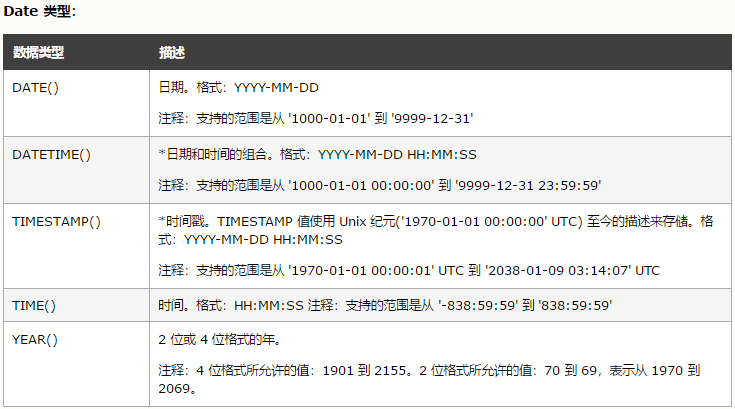约束表示 含义 NOT NULL 本字段不接受NULL值 ``````CREATE TABLE 表名 (   字段名 类型 NOT NULL )`````` UNIQUE 本字段的值是唯一的。一个表可以有多个UNIQUE字段 ``````CREATE TABLE Persons (   字段名 类型 NOT NULL,   UNIQUE (字段) )`````` PRIMARY KEY 主键。字段是唯一的，并且不可以是NULL，每个表有且仅有一个主键 ``````CREATE TABLE 表名 (   字段名 int NOT NULL,   PRIMARY KEY (字段名) )`````` FOREIGN KEY 指向别的表的主键。可以预防表相连关系被破坏 ``````CREATE TABLE table1 (   id_1 int NOT NULL   FOREIGN KEY (id_1) REFERENCES table2(id_2) )`````` CHECK 限制列中值的约束 ``````CREATE TABLE table1 (   sums int NOT NULL,   CHECK (sums>0) )`````` AUTO_INCREMENT 自增 DEFAULT 默认值 ``````CREATE TABLE table1 (   sums int NOT NULL DEFAULT 'haha'  --默认为haha   dtime date DEFAULT GETDATE()  --默认为当前时间 )``````

## create index（创建索引）

``````CREATE INDEX 索引名 ON 表名 (被索引的列名) --添加索引，允许重复索引值
CREATE UNIQUE INDEX 索引名 ON 表名 (被索引的列名) --添加索引，不允许重复索引值``````

## drop（删除）

``````ALTER TABLE 表名 DROP INDEX 索引名  --删除索引
DROP TABLE 表名称  --删除表
DROP DATABASE 数据库名称  --删除库
TRUNCATE TABLE 表名称  --删除表内数据，但是保留表``````

## alter（结构改变）

``````ALTER TABLE 表名 ADD 列名 数据类型    --添加一个字段（列）
ALTER TABLE 表名 DROP COLUMN 列名     --删除一个字段
ALTER TABLE 表名 ALTER COLUMN 列名 数据类型  --修改字段数据类型``````

## group by（组归并）

``````--统计每个stu_name对应的point之和。同stu_name会被合并到一组求和
SELECT stu_name,SUM(point) FROM stu GROUP BY stu_name

--不加GROUP BY会导致SUM(point)的值全部为全表总值``````

## having（过滤）

where语句不能用sum等聚合函数。having可以在sql结果集中筛选出满足条件的结果

``````--例如：筛选1班和2班分数不及格的学生
SELECT stu_name,SUM(point) FROM stu
WHERE class=1 OR class=2
GROUP BY stu_name
HAVING SUM(point)<60``````

## 函数参考

 函数 作用 举例 IFNULL IFNULL(col,a)判断col字段是不是NULL，如果是，则返回a ``````SELECT point*(p_count+IFNULL(n_count,0)) AS result_col_name FROM tb1`````` NOW/CURDATE/CURTIME NOW:当前日期时间 CURDATE:当前日期 CURTIME:当前时间 AVG 求平均 ``````SELECT person FROM tb WHERE point>(SELECT AVG(point) FROM tb) --选择高于均值的`````` COUNT 计数 ``````SELECT COUNT(*) FROM tb --返回行数 SELECT COUNT(col) FROM tb --返回列不为NULL数量 SELECT COUNT(DISTINCT col) FROM tb --返回不同值数量``````